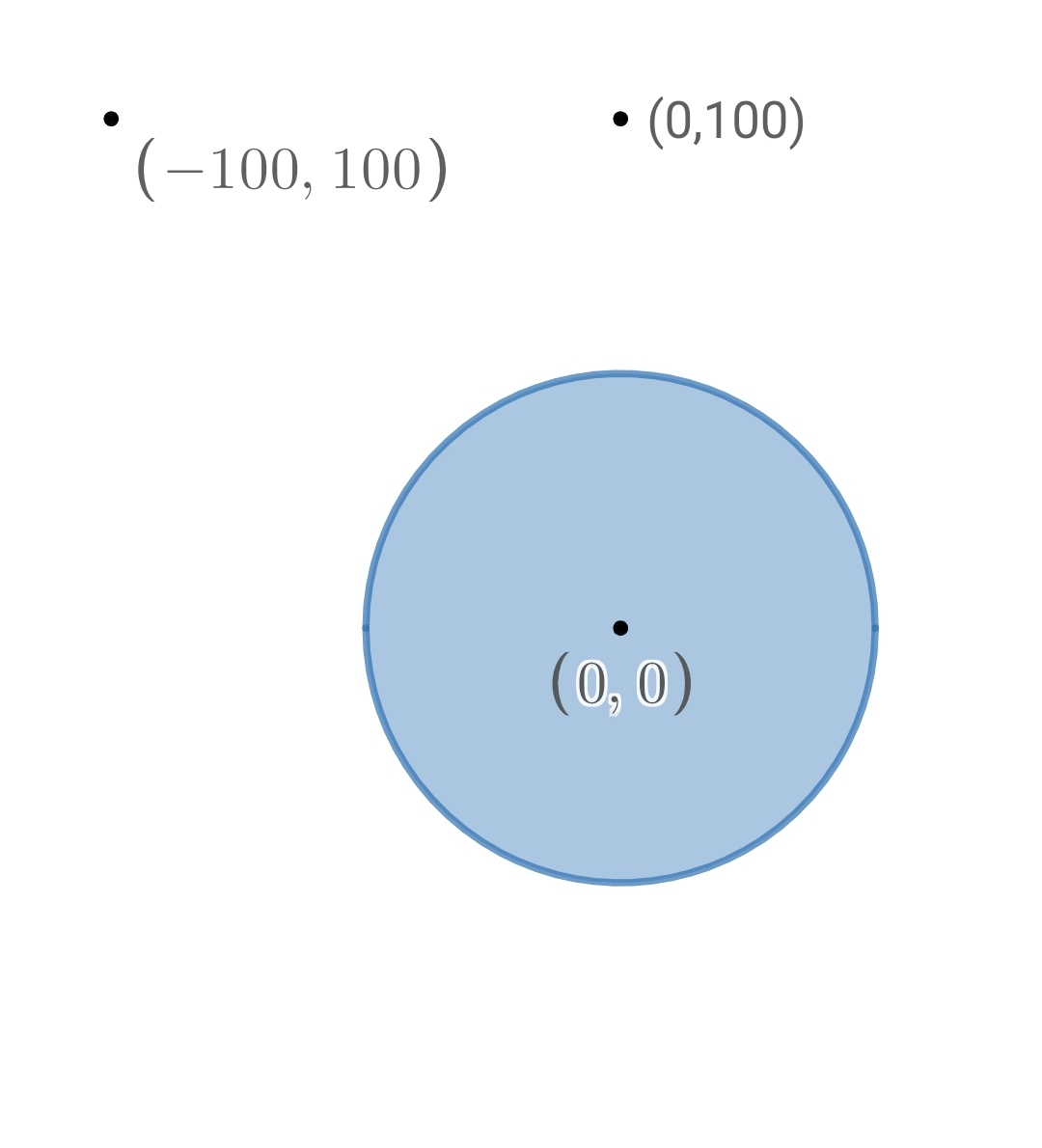# Two points And A Circle Of Life!

Geometry Level 4A person wants to go from point $(-100,100)$ to edge of a circular pond of radius $50$ and center $(0,0)$ to fill water. From there, he goes to point $(0,100)$. You can't swim and so you can't go inside circular pond and only go to it's edge to fill water.

If the length of the shortest path for above process is $l$, Enter answer as $\lfloor l \rfloor$ where $\lfloor . \rfloor$ denotes Floor Function

Try similar problems

All of my problems are original.

×